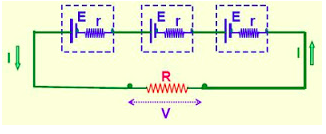Parallel combinations of cell circuits have various paths in between the terminals. All the negative terminals of the cells are connected together whereas positive terminals of the cells are connected together. In a Series combination of cells , there is a single path between the terminals. The positive terminal of the cell are connected to the negative terminal unlike the parallel cell of the other cell in a series combination

## Combination of Cells in Series

Combination of cells in series the cells are connected in such a way that they are joined from end to end to get the same quantity of electricity throughout the cell.Combination of Cells in Series

• In a series combination of cells, the cells are joined end to end so that the same current flows through each cell is identical with the current in the entire arrangement.
• In case if the cells are connected in series the electromotive force of the battery is connected to the sum of the electromotive force of the individual cells.
• The sum of the individual emfs is the electromotive force of the battery.

### Resistance of Cells in Series

• If F is the overall electromotive force (emf)  of the battery combined with n number cells and F1, F2, F3 , Fn are the emfs of individual cells. Then   F1 + F2 +  F3 + …….Fn
• Similarly, if i1, i2, i3, in are the internal resistances of individual cells, then internal resistance of the battery is equal to the sum of the internal resistance of the individual cells i.e. i = i1 + i2+  i3 +  in

### Advantages of Cells Connected in Series

• When Cells are connected in series they give a greater resultant voltage than individual cells.
• Voltage increases if the number of cells increases.
• Series circuits do not overheat easily.

### Disadvantages of Cells Connected in Series

• Even If one component in a series circuit gets damaged, then all the components in the circuit fail because the circuit has been broken.
• The circuit’s resistance is greater when there more components in a series circuit.

## Combination of cells in Parallel

Combinations of cells in parallel are connected in such a way that positive is connected to positive terminal and negative to negative terminal.

Combination of cells in Parallel

• The electromotive force  of the battery is the same as a single cell.
• The external circuit current is divided equally among the cells.
• In a parallel combination, all the positive terminals are connected together and all the negative terminals are connected together.

### Resistance of Cells in Parallel

• If the electromotive force (emf) of each cell is similar, then the emf of the battery combined with ‘n’ numbers of cells connected in parallel will be equal to the emf of each cell. The resultant internal resistance of the combination is,Equivalent EMF/Resistance of Cells in Series and Parallel
• Assuming that  the reciprocal of the total internal resistance is the sum of the reciprocals of the individual internal resistances.
Total ‘emf’ of the battery = E
Total Internal resistance of the battery = r / n
Total resistance of the circuit = (r / n) + R

If R << r/n, Then I = n(E / r) ; If r/n << R, then I = E / R

### Advantages of Cells Connected in Parallel

•  If even one of the cells connected in parallel is damaged, the other cells will not be affected.
•  When the cells are connected in parallel they will not exhaust easily.

### Disadvantages of Cells Connected in Parallel

• The voltage developed does not  increase if we increase the number of cells in the parallel combination.
• The brightness of the bulb connected will not be very high as the output power is based on one cell.